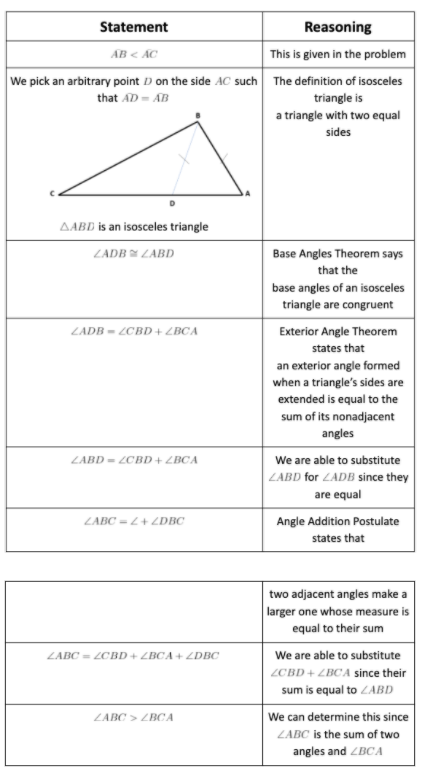# Common Core: High School - Geometry : Prove theorems about triangles.

## Example Questions

### Example Question #1 : Prove Theorems About Triangles.

True or False: The SAS Theorem, ASA Theorem, SSS Theorem, and AA Theorem are all theorems that prove triangles to be congruent.

False

True

False

Explanation:

Three of these theorems do prove triangles to be congruent; SAS (Side-Angle-Side), ASA (Angle-Side-Angle), and SSS (Side-Side-Side).  The AA (Angle-Angle) Theorem states that two triangles with two congruent, corresponding angles are similar NOT necessarily congruent.

### Example Question #4 : Prove Theorems About Triangles.

Which of the following theorems is used only to prove the congruence of two right triangles?

SSS Theorem

SAS Theorem

HL Theorem

ASA Theorem

HL Theorem

Explanation:

While all of these theorems can prove two triangles to be congruent the Hypotenuse-Leg Theorem (HL) is the only theorem out of these that can only prove two right triangles to be congruent. This theorem states that if two right triangles have one congruent leg and a congruent hypotenuse then they are congruent.

### Example Question #1 : Prove Theorems About Triangles.

What is the first step to any proof?

State the given

Draw a picture

There is no set first step

State the given

Explanation:

In order to prove anything, we must first state the given information.  This will allow us to move forward with the proof and make different connections based on the given information.

### Example Question #2 : Prove Theorems About Triangles.

Which of the following is the altitude rule?

The altitude to the leg of a right triangle is the mean proportional between the segments into which it divides the leg.

The altitude to the hypotenuse of a right triangle is the mean proportional between the segments into which it divides the leg.

The altitude to the hypotenuse of a right triangle is the mean proportional between the segments into which it divides the hypotenuse.

The altitude to the leg of a right triangle is the mean proportional between the segments into which it divides the hypotenuse.

The altitude to the hypotenuse of a right triangle is the mean proportional between the segments into which it divides the hypotenuse.

Explanation:

This can be represented by the following equation### Example Question #1 : Prove Theorems About Triangles.

True or False: The Base Angles Converse states that if two base angles are congruent, then their opposite sides are congruent.

False

True

True

Explanation:

Since the Base Angles Theorem states that if two adjacent sides are congruent then their opposite angles are congruent, then its converse assumes that the two base angles are congruent and then their opposite sides are congruent.

### Example Question #10 : Prove Theorems About Triangles.

Consider the following theorem: If two triangles have two pairs of congruent corresponding sides and their included angle is congruent, then these two triangles are congruent.  When proving this theorem, what information do we assume to begin the proof?

Assume that two triangles have two pairs of corresponding congruent sides and their included angle is congruent

Assume that the two triangles are congruent

Assume that two triangles have a pair of corresponding congruent angles

Assume that two triangles have two pairs of corresponding congruent sides

Assume that two triangles have two pairs of corresponding congruent sides and their included angle is congruent

Explanation:

When theorems are presented to you in an “if-then” format, you always assume the entire “if” part.  This allows you to construct an argument that you will be able to prove to be true using the given information.

### Example Question #2 : Prove Theorems About Triangles.

Fill in the blanks below to complete the proof for the HL Theorem.  Use the figure below to find the given information.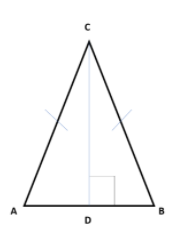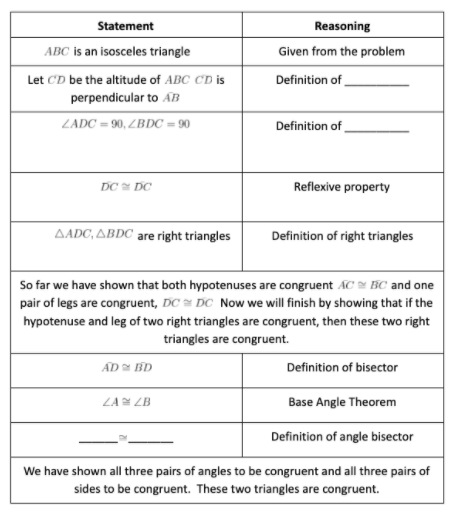perpendicular, altitude,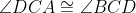altitude, perpendicular,angle bisector, bisector,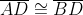altitude, perpendicular,Explanation: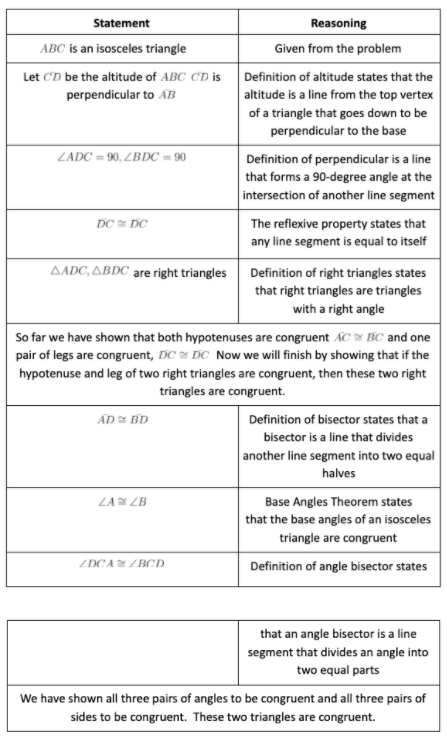### Example Question #5 : Prove Theorems About Triangles.

The triangle inequality theorem states that if you have a triangle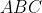then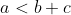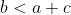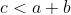Fill in the blanks for the proof of the theorem below using triangle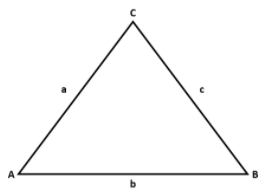To begin this proof we first must let there exist the triangle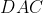, where the length of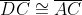and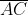is a shared side between the two triangles.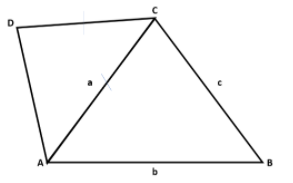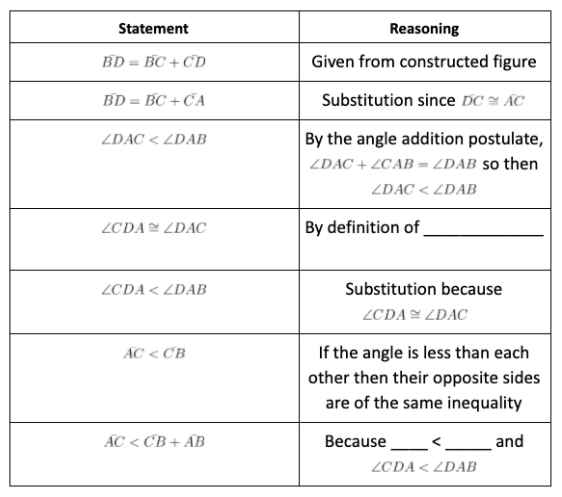obtuse triangle,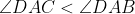isosceles triangle,equilateral triangle,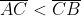isosceles triangle,Explanation:

Follow the detailed proof below for an explanation.

To begin this proof we first must let there exist the triangle, where the length ofandis a shared side between the two triangles.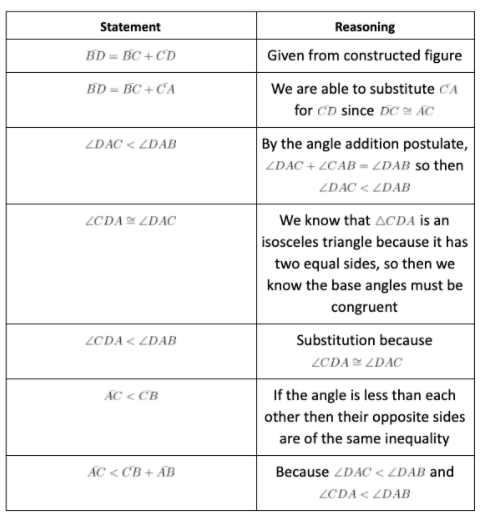### Example Question #3 : Prove Theorems About Triangles.

Fill in the blanks to the proof below.  We are proving that the base angles of isosceles triangles,, are congruent.  This is a proof of the isosceles triangle theorem.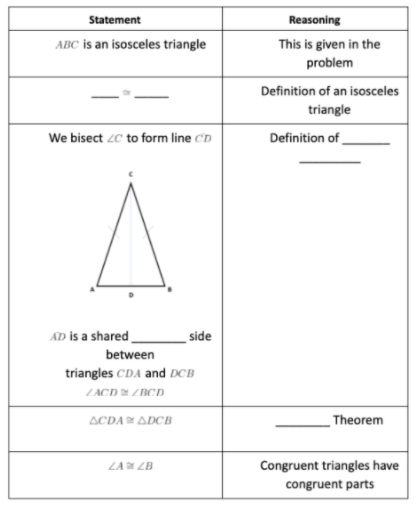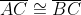, congruent, midpoint, ASA, congruent, angle bisector, SAS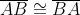, similar, angle bisector, SAS, congruent, angle bisector, SAS

Explanation: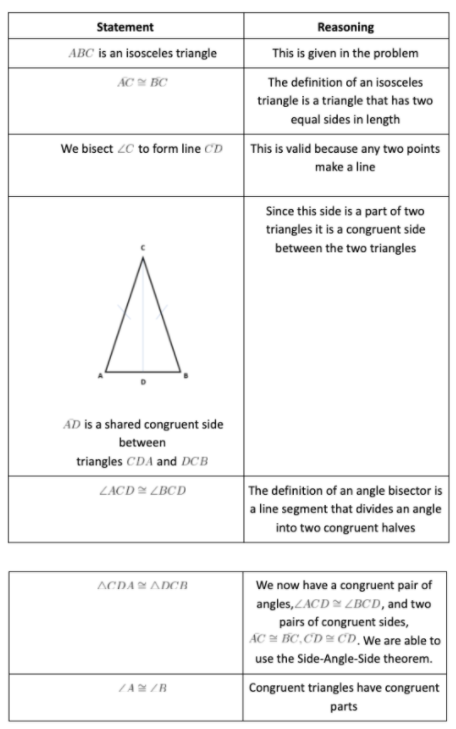### Example Question #3 : Prove Theorems About Triangles.

Prove that if one side of a triangle is longer than another side of the same triangle, then the angle opposite the longer side will be greater than the angle opposite the shorter side.  Use the information that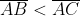for triangle.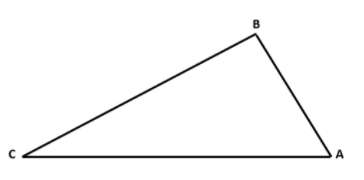Proof: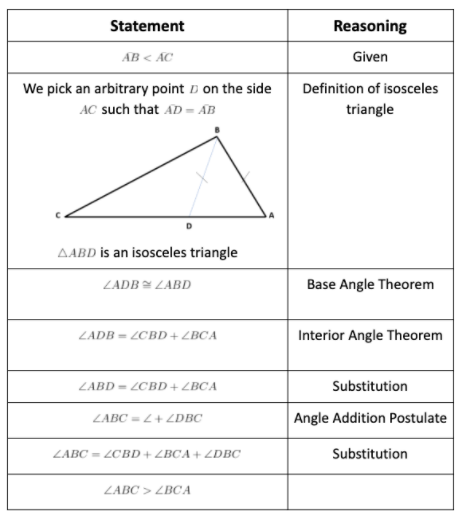Proof: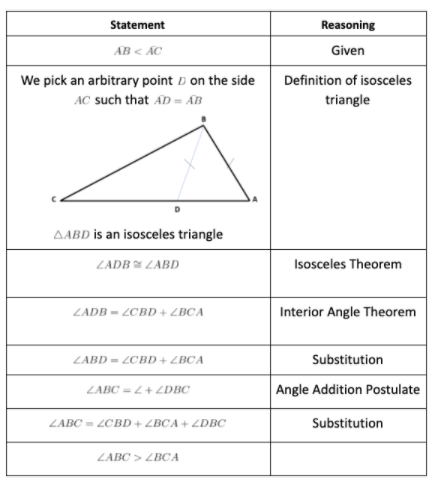Proof: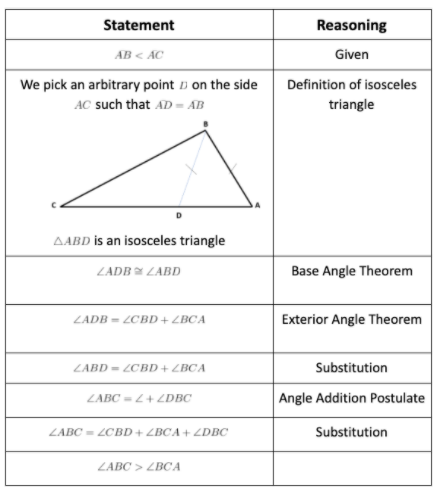Proof:Explanation: